# Precalculus : Find the Unit Vector in the Same Direction as a Given Vector

## Example Questions

### Example Question #1 : Find The Unit Vector In The Same Direction As A Given Vector

Find the unit vector that is in the same direction as the vector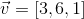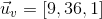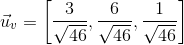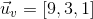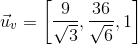Explanation:

To find the unit vector in the same direction as a vector, we divide it by its magnitude.

The magnitude of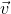is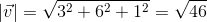.

We divide vectorby its magnitude to get the unit vector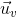: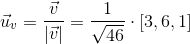orAll unit vectors have a magnitude of, so to verify we are correct: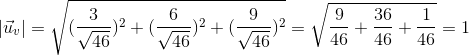### Example Question #1 : Find The Unit Vector In The Same Direction As A Given Vector

A unit vector has length.

Given the vector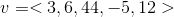find the unit vector in the same direction.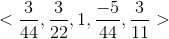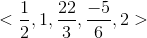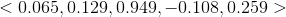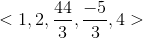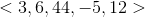Explanation:

First, you must find the length of the vector. This is given by the equation: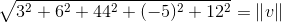Then, dividing the vector by its length gives the unit vector in the same direction.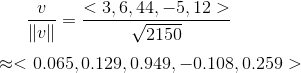### Example Question #3 : Find The Unit Vector In The Same Direction As A Given Vector

Put the vector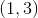in unit vector form.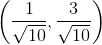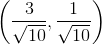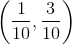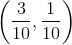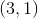Explanation:

To get the unit vector that is in the same direction as the original vector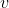, we divide the vector by the magnitude of the vector.

For, the magnitude is: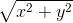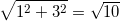.

This means the unit vector in the same direction ofis,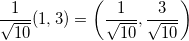### Example Question #1 : Find The Unit Vector In The Same Direction As A Given Vector

Find the unit vector of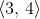.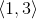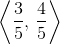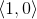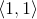Explanation:

In order to find the unit vector u of a given vector v, we follow the formula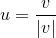Let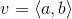The magnitude of v follows the formula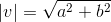.

For this vector in the problem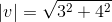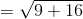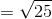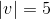.

Following the unit vector formula and substituting for the vector and magnitude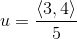.

As such,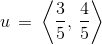.

### Example Question #1 : Find The Unit Vector In The Same Direction As A Given Vector

Find the unit vector of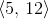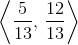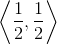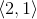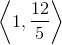Explanation:

In order to find the unit vector u of a given vector v, we follow the formulaLetThe magnitude of v follows the formulaFor this vector in the problem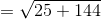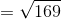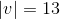Following the unit vector formula and substituting for the vector and magnitude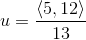As such,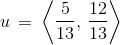### All Precalculus Resources